## RS Aggarwal Solutions Class 10 Chapter 13 Constructions Ex 13a

These Solutions are part of RS Aggarwal Solutions Class 10. Here we have given RS Aggarwal Solutions Class 10 Chapter 13 Constructions Ex 13a.

Other Exercises

Question 1.
Solution: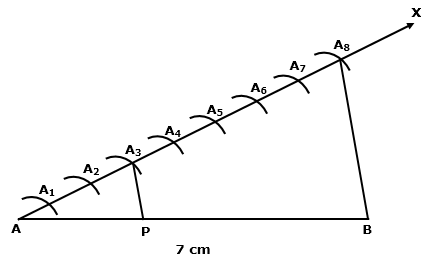Question 2.
Solution: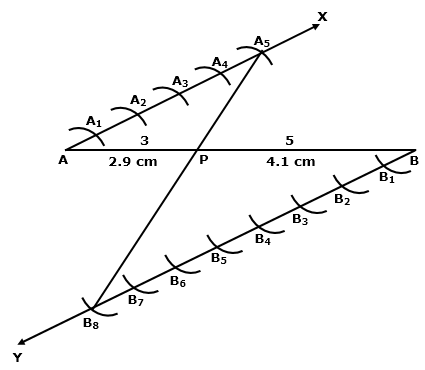Question 3.
Solution: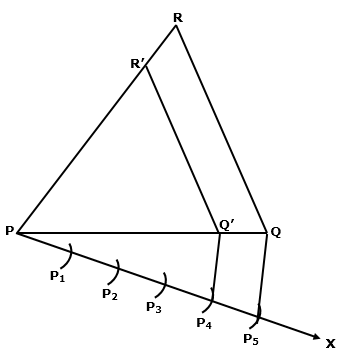Question 4.
Solution:Question 5.
Solution: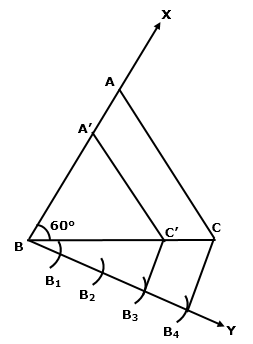Question 6.
Solution:Question 7.
Solution: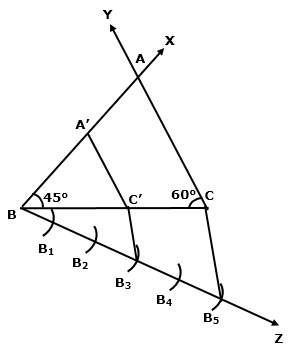Question 8.
Solution:
BC will be divided in the ratio 3 : 4

Question 9.
Solution: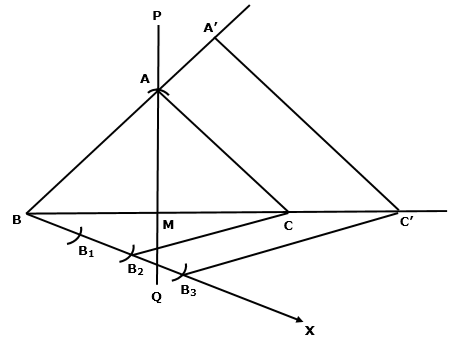Question 10.
Solution: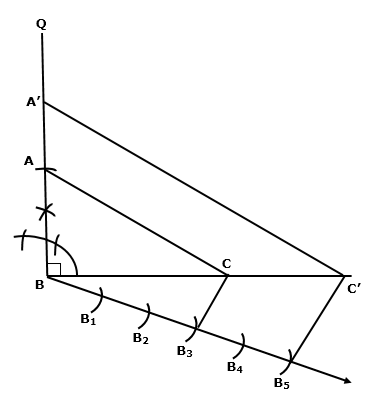Hope given RS Aggarwal Solutions Class 10 Chapter 13 Constructions Ex 13a are helpful to complete your math homework.

If you have any doubts, please comment below. Learn Insta try to provide online math tutoring for you.